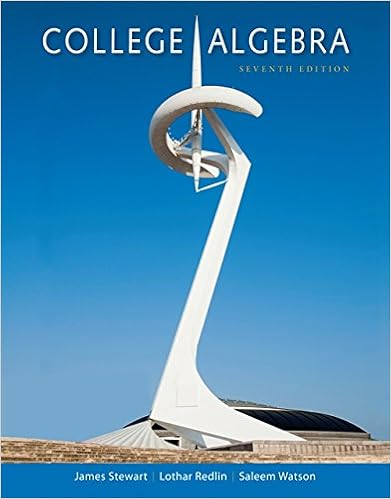# End is hinged calculate the crippling load by

• Homework Help
• 520

This preview shows page 195 - 204 out of 520 pages.

##### We have textbook solutions for you!
The document you are viewing contains questions related to this textbook.The document you are viewing contains questions related to this textbook.
Chapter 5 / Exercise 50
College Algebra
Redlin/StewartExpert Verified
end is hinged. Calculate the crippling load by Rankine’s formula. Compare this with the load obtained by Euler’s formula. For what length of this strut will the two formulae give the same crippling load for the joist? Area of cross-section, A = 5,490 mm 2 I xx = 9,874.6 × 10 4 mm 4 I yy = 510.8 × 10 4 mm 2 E = 210 kN/mm 2 σ c = 320 N/mm 2 a = for both hinged ends. Solution I yy < I xx L = 6 m
##### We have textbook solutions for you!
The document you are viewing contains questions related to this textbook.The document you are viewing contains questions related to this textbook.
Chapter 5 / Exercise 50
College Algebra
Redlin/StewartExpert Verified
(one end is fixed and the other end is hinged) Euler’s buckling load. Rankine’s load, Equating two loads, Putting the values,
L = 8.48 × 103m, for this length both P e and P R are equal L = 8.48 m Problem 14.6 A round bar in a vertical position is clamped at the lower end and is free at the other end. The effective length is 2 m. If a horizontal force of 400 N at the top produces a horizontal deflection of 15 mm, what is the axial buckling load for the bar under the given conditions? Figure 14.22 Problem 14.6 Solution
The bar is fixed as a cantilever beam (as shown Fig. 14.22 ). Deflection at the top Buckling load, P e = π 2 × EI /4 L 2 , as one end is fixed and the other end is free Problem 14.7 A 3-m-long straight steel bar of a rectangular section of 30 mm × 20 mm is used as a strut with both the ends hinged. Assuming that Euler’s formula is applicable and the material attains its yield strength at the time of buckling, determine the central deflection. E = 210 kN/mm 2 . Yield strength of steel is 320 N/mm 2 . Solution Section 30 × 20 mm 2 , A = 600 mm 2 E = 2,10,000 N/mm 2
L = 3,000 mm (both the ends are hinged) Euler’s buckling load, Say, central deflection, y max . Problem 14.8 A steel column of a hollow circular section of 200 mm external diameter, 160 mm internal diameter and 7-m-long has to take a 20-kN load at an eccentricity of 30 mm from the geometrical axis. If the ends are fixed, calculate the maximum and minimum stress intensities induced in the section, taking E = 210 kN/mm 2 . Moreover, calculate the maximum permissible eccentricity so that no tension is induced any where in the section. Solution Section
D = 200 mm d = 160 mm A = (200 2 − 160 2 ) = 1.131 × 10 4 mm 2 I = (200 4 − 160 4 ) = 0.4637 × 10 8 mm 4 P = 200 kN E = 2,10,000 N/mm 2 L = 7 m Ends are fixed, L e = L /2 = 3.5 m = 3,500 mm y c = 100 mm Eccentricity, e = 30 mm
For no tension Eccentricity, = 34.3 mm Key Points to Remember o Euler’s buckling load, P e = π 2 EI min / L e 2 where I min = minimum moment of inertia L e = equivalent length of a column depending upon end conditions.
o Euler’s formula is applicable for the slenderness ratio , where σ c is ultimate compressive strength of the material. o Higher-order differential equation o Solution, y = C 1 sin kx + C 2 cos kx + C 3 x + C 4 using different end conditions, the buckling load is determined. o Rankine’s load = where σ c = crushing strength of column L e = equivalent length, k min = minimum radius of gyration o Johnson’s parabolic formula for working stress where σ c = allowable stress in compressive taking into account FOS b = constant o Eccentrically loaded column where P = axial applied load Z = section modulus e = eccentricity I = moment of inertia o Professor Perry’s formula
where σ = maximum stress allowed σ 0 = P / A , applied load/area σ e = P e / A , Euler’s load/area e = eccentricity y c = distance of extreme layer in compression from neutral layer o
•••